Number Representation Real numbers Converting denary to floating

• Slides: 7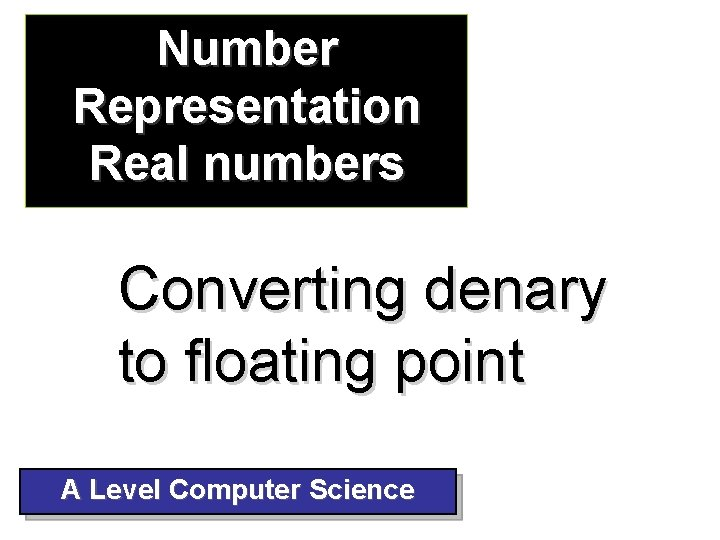Number Representation Real numbers Converting denary to floating point A Level Computer Science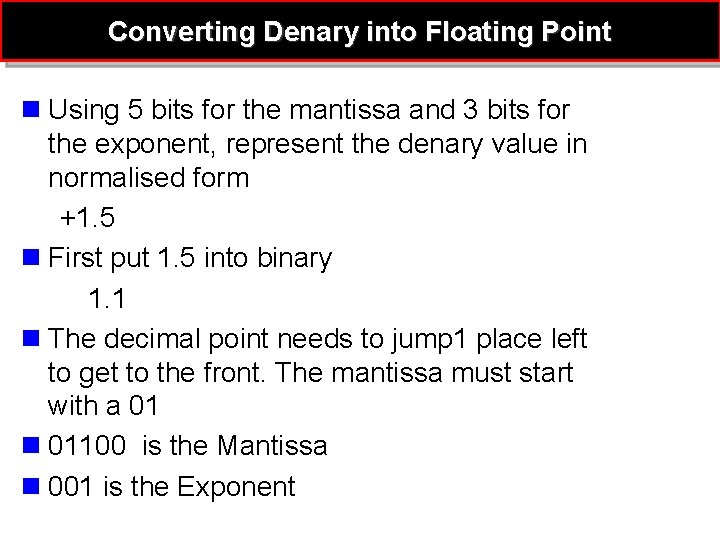Converting Denary into Floating Point n Using 5 bits for the mantissa and 3 bits for the exponent, represent the denary value in normalised form +1. 5 n First put 1. 5 into binary 1. 1 n The decimal point needs to jump 1 place left to get to the front. The mantissa must start with a 01 n 01100 is the Mantissa n 001 is the Exponent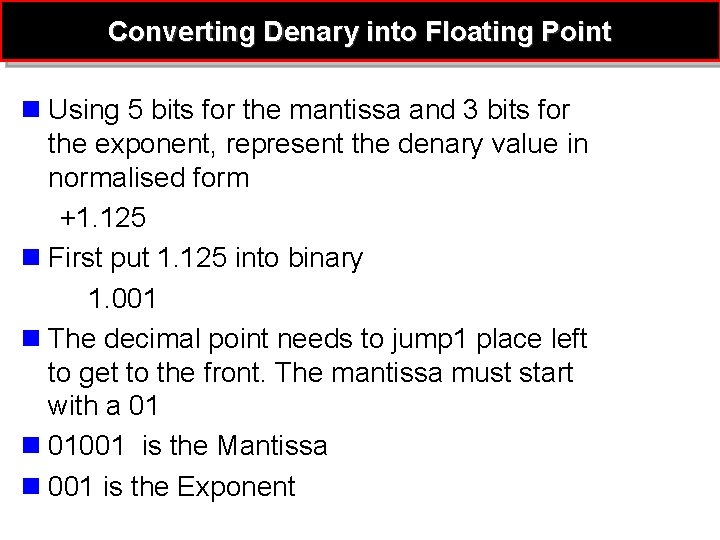Converting Denary into Floating Point n Using 5 bits for the mantissa and 3 bits for the exponent, represent the denary value in normalised form +1. 125 n First put 1. 125 into binary 1. 001 n The decimal point needs to jump 1 place left to get to the front. The mantissa must start with a 01 n 01001 is the Mantissa n 001 is the Exponent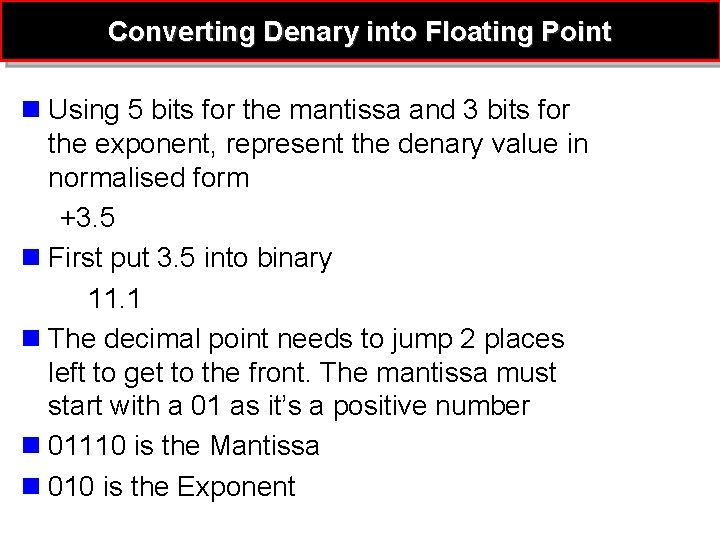Converting Denary into Floating Point n Using 5 bits for the mantissa and 3 bits for the exponent, represent the denary value in normalised form +3. 5 n First put 3. 5 into binary 11. 1 n The decimal point needs to jump 2 places left to get to the front. The mantissa must start with a 01 as it’s a positive number n 01110 is the Mantissa n 010 is the Exponent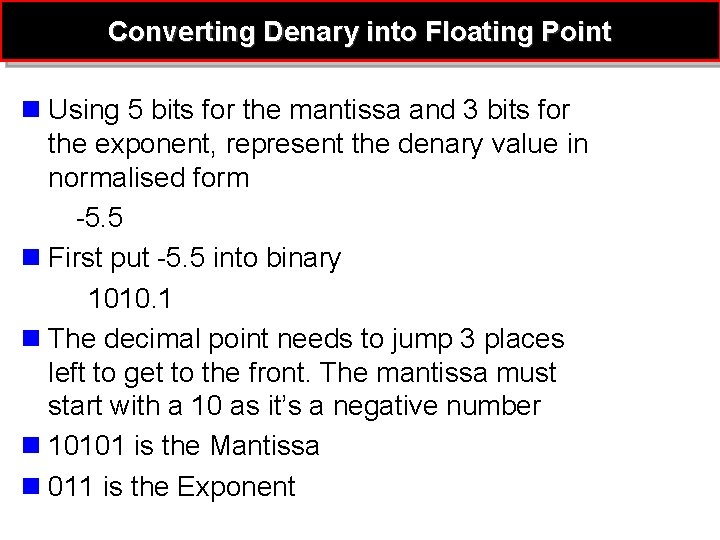Converting Denary into Floating Point n Using 5 bits for the mantissa and 3 bits for the exponent, represent the denary value in normalised form -5. 5 n First put -5. 5 into binary 1010. 1 n The decimal point needs to jump 3 places left to get to the front. The mantissa must start with a 10 as it’s a negative number n 10101 is the Mantissa n 011 is the Exponent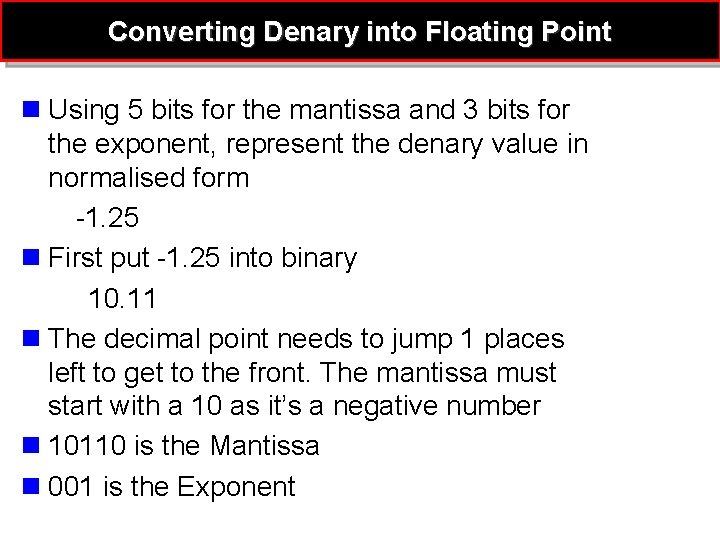Converting Denary into Floating Point n Using 5 bits for the mantissa and 3 bits for the exponent, represent the denary value in normalised form -1. 25 n First put -1. 25 into binary 10. 11 n The decimal point needs to jump 1 places left to get to the front. The mantissa must start with a 10 as it’s a negative number n 10110 is the Mantissa n 001 is the Exponent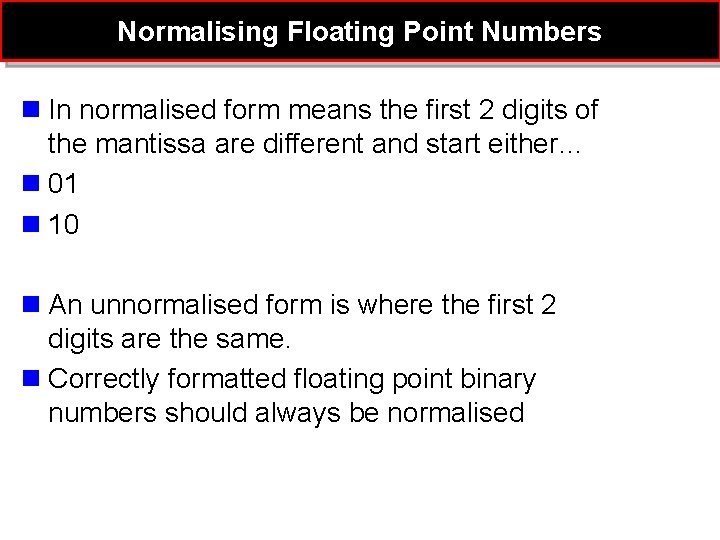Normalising Floating Point Numbers n In normalised form means the first 2 digits of the mantissa are different and start either… n 01 n 10 n An unnormalised form is where the first 2 digits are the same. n Correctly formatted floating point binary numbers should always be normalised